Goseeko blog# What is successive differentiation?

Successive differentiation is the process in which we can differentiate the function successively multiple times and the derivative we find is called the successive derivative. Successive differentiation is very important in engineering and scientific applications.

It is the process of differentiating the given function simultaneously many times and  the result  obtained are  called successive  derivatives.

Let y = f(x) be a differentiable function.

First derivative is denoted by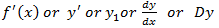Second derivative is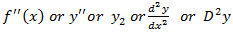Third derivative is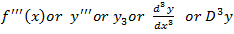Similarly the nth derivative is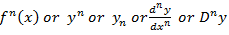## Successive differentiation

The successive differential coefficients of y are denoted as follows-

The differential coefficient is-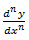Other notations to denote n’th differential coefficients-

The process of applying differentiation again and again is called successive differentiation.

nth derivative of standard functions-

1. nth derivative of   –

Suppose y =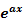,

Differentiate with respect to x successively, we get

For n times differentiation, we get-

So we can say that its n’th derivative will be

2. nth derivative of log(ax + b)-

Suppose y = log (ax + b)

Differentiate with respect to x successively, we get

For n times differentiation, we get-

So we can say that its n’th derivative will be

3. nth derivative of  (ax +b)^m-

Suppose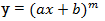Differentiate with respect to x successively, we get

For n times differentiation, we get-

So we can say that its n’th derivative will be

4.  nth derivative of sin(ax + b)-

Suppose y = sin(ax + b)

Differentiate with respect to x successively, we get

For n times differentiation, we get-

So we can say that its n’th derivative will be

Similarly we can find the ‘n’ derivatives of such functions.

Standard results-

Example: Find the nth  derivative of

Sol. Here we have-

Suppose,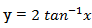First derivative-

Here

Let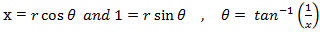So that

Which is the n’th derivative of the given function.

Example: Find cos x cos 2x cos 3x.

Sol:

So that-

n’th derivative-

Example: Find the nth derivative of the following function-

Sol.  Partial fraction of the function y after splitting-

Suppose x – 1 = z, then

Example: Find nth derivative of the given function:

Sol.   We are given-

factorize the denominator-

nth derivative will be-

Which is the derivative of the given function.

Interested in learning about similar topics? Here are a few hand-picked blogs for you!